• 一、非确定性有限自动机 ( NFA ) 转为 确定性有限自动机 ( DFA )、 二、转换方法与要点


文章目录
一、非确定性有限自动机 ( NFA ) 转为 确定性有限自动机 ( DFA )二、转换方法与要点

一、非确定性有限自动机 ( NFA ) 转为 确定性有限自动机 ( DFA )

确定性有限自动机 ( DFA ) 与 非确定性有限自动机 ( NFA ) 之间是相互等价的 ;
确定性的有限自动机 ( DFA ) 可以 看作是非确定性有限自动机 ( NFA ) ;

确定性有限自动机 给定一个输入 , 其输出时唯一的 ;
非确定性有限自动机的定义 包含 确定性有限自动机的 定义中 ;

NFA 的后继状态 可以是

0

0

个 ,

1

1

个 或 多个 , DFA 每个状态只能有

1

1

个后继状态 ;
确定性有限自动机 ( DFA ) 就是 特殊的 非确定性有限自动机 ( NFA ) ;
可以证明非确定性有限自动机 ( NFA ) , 必定有一个 确定性有限自动机 ( DFA ) 与其等价 ;

参考博客 :
【计算理论】非确定性有限自动机 ( 计算过程 | 计算树 | 确定可接受字符串 | 设计非确定性有限自动机 | 空字符 )【计算理论】非确定性有限自动机 ( NFA ) 转换成 确定性有限自动机 ( DFA )

二、转换方法与要点

1. 转换方法 :
从 起始状态 开始推演运行 ,
列出所有的 分支步骤 ,
注意 计算分叉节点 , 会产生多个后续状态 ,
此时就生成了 新的状态 ,
这些新的状态就是非确定性有限自动机 转换成的 确定性有限自动机的 新状态 ;

2. 转换要点 :
① 新状态生成时机 : 有两种情况会出现计算分支 ,
情况一 : 状态有

ε

\rm \varepsilon

无条件跳转 , 如下图的

1

1

状态 , 会无条件跳转到

3

3

状态 , 此时就会出现两个后续状态

{

1

,

3

}

\rm \{ 1, 3 \}

,
情况二 : 读取同样的字符 , 有两个后继状态 , 如

2

2

状态下读取

a

\rm a

字符 , 会跳转到

2

2

状态和

3

3

状态 , 因此其后继状态是

{

2

,

3

}

\rm \{ 2, 3 \}

,
情况三 : 计算出现新状态后 , 新状态的后继状态 , 一般也是一个集合 , 当计算

{

1

,

3

}

\rm \{ 1, 3 \}

的后续状态时 , 会分别计算集合中的两个状态分别读取

a

\rm a

字符的后继状态 , 取并集 ;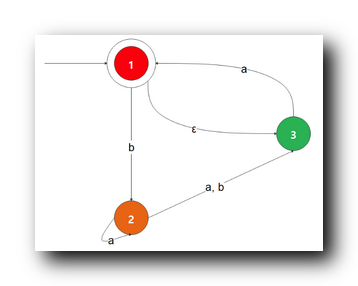② 新状态的计算机制 : 如果生成了一个新的状态 ,

{

1

,

3

}

\rm \{ 1, 3 \}

, 如果要计算其后继状态时 , 就需要分别计算

1

1

和

3

3

的后继状态, 然后取并集 ;
③ 空集 : 在推演计算时 , 有可能会出现空集 , 如

{

3

}

\rm \{ 3 \}

状态读取

b

\rm b

字符的后继状态没有 , 就是空集 ;

3. 接受状态 : 如果最终的 DFA 的新状态集合中 , 包含 NFA 的接受状态 , 那么该新状态就是接受状态 ;

4. 空集 : 如果其中有空集 , 那么将空集也当做一个状态 , 空集状态下读取任何字符都是空集 ;

5 . 后继状态有

ε

\rm \varepsilon

无条件跳转 : 如果读取字符后跳转的 后继状态有

ε

\rm \varepsilon

无条件跳转 , 则该后继状态会是两个状态的集合 , 如 :

{

3

}

\rm \{ 3 \}

状态读取

a

\rm a

字符跳转到

1

1

状态 , 而

1

1

状态无条件跳转到

3

3

状态 , 则后继状态是

{

1

,

3

}

\rm \{1, 3\}

;

参考博客 :
【计算理论】非确定性有限自动机 ( 计算过程 | 计算树 | 确定可接受字符串 | 设计非确定性有限自动机 | 空字符 )【计算理论】非确定性有限自动机 ( NFA ) 转换成 确定性有限自动机 ( DFA )
展开全文计算理论
• 非确定性有限自动机 NFA 转为确定性有限自动机 DFA 示例


文章目录
一、NFA 转 DFA 示例 1二、NFA 转 DFA 示例 2三、NFA 转 DFA 示例 3

一、NFA 转 DFA 示例 1

将下图的 非确定性有限自动机 NFA 转为确定性有限自动机 DFA ;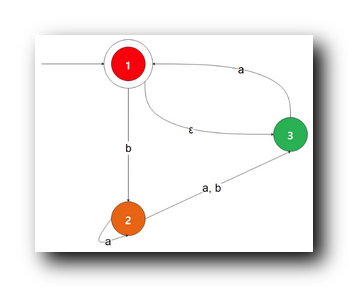NFA 的状态集

{

1

,

2

,

3

}

\rm \{ 1,2,3 \}

, 字符集

{

a

,

b

}

\rm \{ a,b \}

;

从 起始状态

1

1

开始分析 , 读取

ε

\rm \varepsilon

无条件跳转到

3

3

, 这里形成了新的状态

{

1

,

3

}

\rm \{1, 3\}

, 写到下面表格中 ;

{

1

,

3

}

\rm \{1, 3\}

状态 下读取

a

\rm a

字符结果是

{

1

,

3

}

\rm \{1, 3\}

, 读取

b

\rm b

字符结果是

{

2

}

\{2\}

, 上述分别是 NFA 下两个状态读取字符的后继状态取并集 ; 将新状态写到表格中 , 然后分析新状态 ;

{

2

}

\{2\}

状态下读取读取

a

\rm a

字符结果是

{

2

,

3

}

\{2,3\}

, 读取

b

\rm b

字符结果是

{

3

}

\{3\}

, 上述分别是 NFA 下两个状态读取字符的后继状态取并集 ; 将新状态写到表格中 , 然后分析新状态 ;

{

2

,

3

}

\{2,3\}

状态下读取读取

a

\rm a

字符结果是

{

1

,

2

,

3

}

\{1, 2,3\}

, 读取

b

\rm b

字符结果是

{

3

}

\{3\}

, 上述分别是 NFA 下两个状态读取字符的后继状态取并集 ; 将新状态写到表格中 , 然后分析新状态 ;

{

3

}

\{3\}

状态下读取读取

a

\rm a

字符结果是

{

1

,

3

}

\{1,3\}

, 读取

b

\rm b

字符结果是

{

∅

}

\{ \varnothing \}

, 上述分别是 NFA 下两个状态读取字符的后继状态取并集 ; 将新状态写到表格中 , 然后分析新状态 ;

{

1

,

2

,

3

}

\{1, 2,3\}

状态下读取读取

a

\rm a

字符结果是

{

1

,

2

,

3

}

\{1, 2,3\}

, 读取

b

\rm b

字符结果是

{

2

,

3

}

\{2, 3\}

, 上述分别是 NFA 下两个状态读取字符的后继状态取并集 ; 将新状态写到表格中 , 然后分析新状态 ;

{

∅

}

\{ \varnothing \}

状态下读取读取

a

\rm a

字符结果是

{

∅

}

\{ \varnothing \}

, 读取

b

\rm b

字符结果是

{

∅

}

\{ \varnothing \}

, 上述分别是 NFA 下两个状态读取字符的后继状态取并集 ;

a

a

b

b

{

1

,

3

}

\{1, 3 \}

{

1

,

3

}

\{1 , 3\}

{

2

}

\{2\}

{

2

}

\{2\}

{

2

,

3

}

\{2,3\}

{

3

}

\{3\}

{

2

,

3

}

\{2,3\}

{

1

,

2

,

3

}

\{1,2,3\}

{

3

}

\{3\}

{

3

}

\{3\}

{

1

,

3

}

\{1,3\}

{

∅

}

\{\varnothing \}

{

1

,

2

,

3

}

\{1,2,3\}

{

1

,

2

,

3

}

\{1,2,3\}

{

2

,

3

}

\{2,3\}

{

∅

}

\{\varnothing \}

{

∅

}

\{\varnothing \}

{

∅

}

\{\varnothing \}

凡是 包含 NFA 中接受状态

1

1

的新状态 都是 接受状态 ;

{

1

,

3

}

\{1, 3 \}

和

{

1

,

2

,

3

}

\{1, 2, 3 \}

都是接受状态 , 画图时都是 双圈 ;
空集

{

∅

}

\{\varnothing \}

状态 , 接受任何字符都是空集

{

∅

}

\{\varnothing \}

;

最终的 DFA 如下 :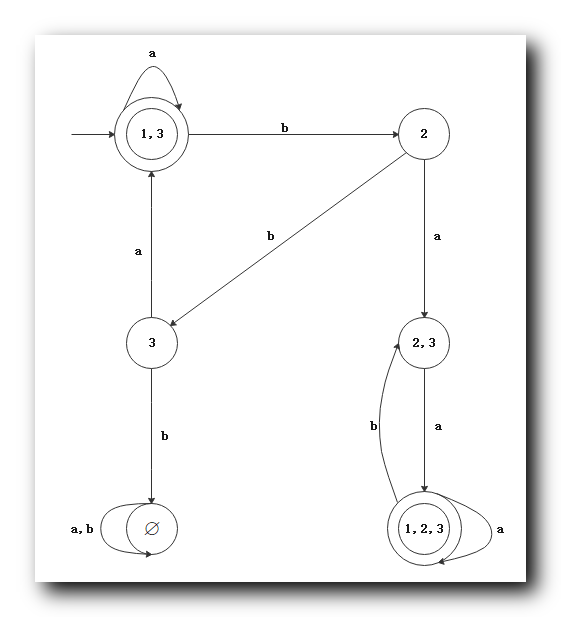详细推理过程 : 【计算理论】非确定性有限自动机 ( NFA ) 转换成 确定性有限自动机 ( DFA )

二、NFA 转 DFA 示例 2

将下图的 非确定性有限自动机 NFA 转为确定性有限自动机 DFA ;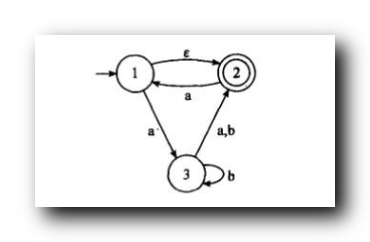NFA 的状态集

{

1

,

2

,

3

}

\rm \{ 1,2,3 \}

, 字符集

{

a

,

b

}

\rm \{ a,b \}

;

从 起始状态

1

1

开始分析 , 读取

ε

\rm \varepsilon

无条件跳转到

2

2

, 这里形成了新的状态

{

1

,

2

}

\rm \{1, 2\}

, 写到下面表格中 ;

{

1

,

2

}

\rm \{1, 2\}

状态 下读取

a

\rm a

字符结果是

{

1

,

2

,

3

}

\rm \{1, 2,3\}

, 读取

b

\rm b

字符结果是

{

∅

}

\{\varnothing \}

;

{

1

,

2

,

3

}

\rm \{1, 2, 3\}

状态 下读取

a

\rm a

字符结果是

{

1

,

2

,

3

}

\rm \{1, 2,3\}

, 读取

b

\rm b

字符结果是

{

2

,

3

}

\{2, 3\}

;

{

2

,

3

}

\rm \{ 2, 3\}

状态 下读取

a

\rm a

字符结果是

{

1

,

2

}

\rm \{1, 2\}

, 读取

b

\rm b

字符结果是

{

2

,

3

}

\{2, 3\}

;

a

a

b

b

{

1

,

2

}

\{1, 2 \}

{

1

,

2

,

3

}

\{1 , 2, 3\}

{

∅

}

\{ \varnothing \}

{

1

,

2

,

3

}

\{1 , 2, 3\}

{

2

,

3

}

\{2,3\}

{

2

,

3

}

\{2,3\}

{

2

,

3

}

\{2,3\}

{

1

,

2

}

\{1,2\}

{

2

,

3

}

\{2,3\}

{

∅

}

\{\varnothing \}

{

∅

}

\{\varnothing \}

{

∅

}

\{\varnothing \}

凡是 包含 NFA 中接受状态

2

2

的新状态 都是 接受状态 ;

{

1

,

2

}

\{1, 2 \}

,

{

2

,

3

}

\{2, 3 \}

和

{

1

,

2

,

3

}

\{1, 2, 3 \}

都是接受状态 , 画图时都是 双圈 ;
空集

{

∅

}

\{\varnothing \}

状态 , 接受任何字符都是空集

{

∅

}

\{\varnothing \}

;

最终的 DFA 如下 :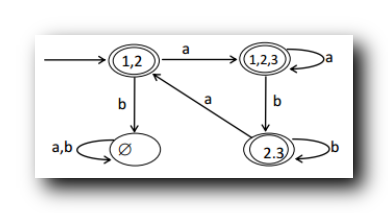三、NFA 转 DFA 示例 3

将下图的 非确定性有限自动机 NFA 转为确定性有限自动机 DFA ;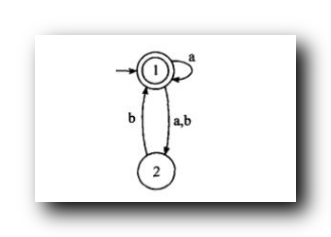NFA 的状态集

{

1

,

2

}

\rm \{ 1,2 \}

, 字符集

{

a

,

b

}

\rm \{ a,b \}

;

从 起始状态

1

1

开始分析 ,

{

1

}

\rm \{1\}

状态 下读取

a

\rm a

字符结果是

{

1

,

2

}

\rm \{1, 2\}

, 读取

b

\rm b

字符结果是

{

2

}

\{ 2 \}

;

{

1

,

2

}

\rm \{1, 2\}

状态 下读取

a

\rm a

字符结果是

{

1

,

2

}

\rm \{1, 2\}

, 读取

b

\rm b

字符结果是

{

1

,

2

}

\{1, 2 \}

;

{

2

}

\rm \{2\}

状态 下读取

a

\rm a

字符结果是

{

∅

}

\{ \varnothing \}

, 读取

b

\rm b

字符结果是

{

1

}

\{1\}

;

a

a

b

b

{

1

}

\{1 \}

{

1

,

2

}

\{1 , 2\}

{

2

}

\{ 2 \}

{

1

,

2

}

\{1 , 2\}

{

1

,

2

}

\{1, 2\}

{

1

,

2

}

\{1,2\}

{

2

}

\{2\}

{

∅

}

\{ \varnothing \}

{

1

}

\{1\}

{

∅

}

\{\varnothing \}

{

∅

}

\{\varnothing \}

{

∅

}

\{\varnothing \}

凡是 包含 NFA 中接受状态

1

1

的新状态 都是 接受状态 ;

{

1

}

\{1\}

和

{

1

,

2

}

\{1, 2 \}

都是接受状态 , 画图时都是 双圈 ;
空集

{

∅

}

\{\varnothing \}

状态 , 接受任何字符都是空集

{

∅

}

\{\varnothing \}

;

最终的 DFA 如下 :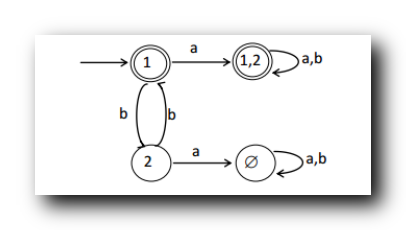展开全文计算理论 自动机
• 确定性有限自动机 ( DFA ) 与 非确定性有限自动机 ( NFA ) 之间是相互等价的 ; 确定性的有限自动机 ( DFA ) 可以 看作是非确定性有限自动机 ( NFA ) ; 确定性有限自动机 给定一个输入 , 其输出时唯一的 ; 非确定性...


文章目录
一、非确定性有限自动机 组成部分二、确定性有限自动机 ( DFA ) 与 非确定性有限自动机 ( NFA ) 等价三、非确定性有限自动机 ( NFA ) 转为 确定性有限自动机 ( DFA ) 示例四、NFA 转 DFA ( 1 ) 开始状态 读取

a

a

字符 后继状态分析五、NFA 转 DFA ( 2 ) 开始状态 读取

b

b

字符 后继状态分析六、NFA 转 DFA ( 3 ) 选取第二个状态进行分析七、NFA 转 DFA ( 4 )

2

2

状态读取

a

a

字符后续状态分析八、NFA 转 DFA ( 5 )

2

2

状态读取

b

b

字符后续状态分析九、NFA 转 DFA ( 6 ) 选取后续需要分析的状态十、NFA 转 DFA ( 7 )

{

2

,

3

}

\{2,3\}

状态 读取

a

a

字符 后继状态分析十一、NFA 转 DFA ( 8 )

{

2

,

3

}

\{2,3\}

状态 读取

b

b

字符 后继状态分析十二、NFA 转 DFA ( 9 ) 选取后续需要分析的状态十三、NFA 转 DFA ( 10 )

{

3

}

\{3\}

状态 读取

a

a

字符 后继状态分析十四、NFA 转 DFA ( 11 )

{

3

}

\{3\}

状态 读取

b

b

字符 后继状态分析十五、NFA 转 DFA ( 12 )

{

1

,

2

,

3

}

\{1,2,3\}

状态 读取

a

a

字符 后继状态分析十六、NFA 转 DFA ( 13 )

{

1

,

2

,

3

}

\{1,2,3\}

状态 读取

b

b

字符 后继状态分析十七、NFA 转 DFA ( 14 ) 结果分析

一、非确定性有限自动机 组成部分

非确定性有限自动机 : Nondeterministic Finite Automaton , NFA ;

①

Q

Q

状态集 : 有限个状态 ;
②

Σ

\Sigma

字母表 : 有限个字符集 , 长度有限的字符串 ;
③

δ

\delta

转移函数 ( 指令集 ) :

δ

\delta

称为转移函数 ; 基于当前的 自动机 的某个状态 , 将字符集 输入到自动机中 , 该自动机转换成一个或多个状态 , 这个转换就是通过

δ

\delta

转换函数进行的 , 使用公式描述

Q

×

Σ

ε

→

2

Q

Q \times \Sigma_{\varepsilon} \to 2^Q

;
④

q

0

q_0

起始状态 : 是自动机的开始状态 ;
⑤

F

F

接受状态集 :

F

F

是 可接受状态 , 是

Q

Q

的子集 , 记做

F

⊆

Q

F \subseteq Q

, 与

F

F

可接受状态相对的是不可接受状态 ;

二、确定性有限自动机 ( DFA ) 与 非确定性有限自动机 ( NFA ) 等价

确定性有限自动机 ( DFA ) 与 非确定性有限自动机 ( NFA ) 之间是相互等价的 ;

确定性的有限自动机 ( DFA ) 可以 看作是非确定性有限自动机 ( NFA ) ;

确定性有限自动机 给定一个输入 , 其输出时唯一的 ;
非确定性有限自动机的定义 包含 确定性有限自动机的 定义中 ;

NFA 的后继状态 可以是

0

0

个 ,

1

1

个 或 多个 , DFA 每个状态只能有

1

1

个后继状态 ;
确定性有限自动机 ( DFA ) 就是 特殊的 非确定性有限自动机 ( NFA ) ;

可以证明非确定性有限自动机 ( NFA ) , 必定有一个 确定性有限自动机 ( DFA ) 与其等价 ;

三、非确定性有限自动机 ( NFA ) 转为 确定性有限自动机 ( DFA ) 示例

正确的图片 :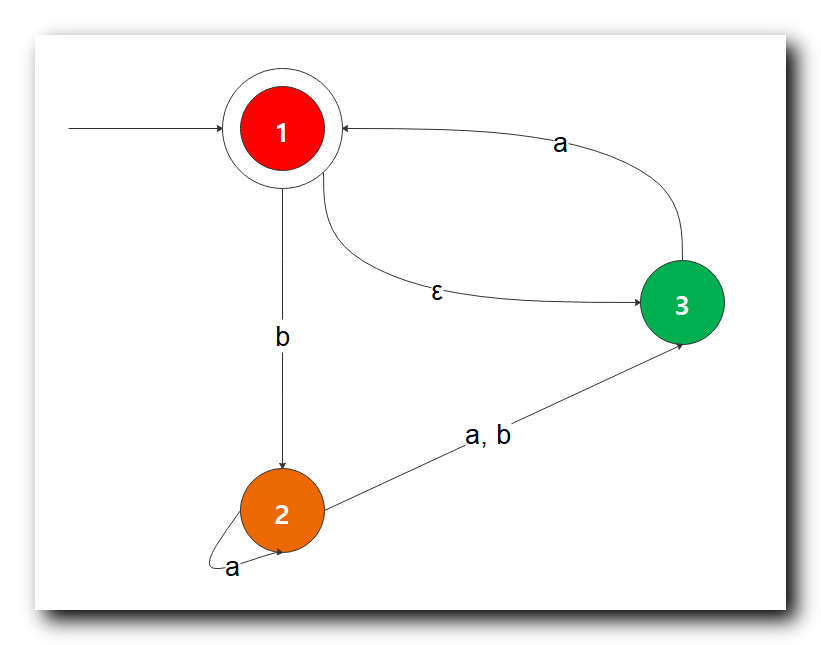下图绘制错误 , 暂时作废 , 看第一张图片 ;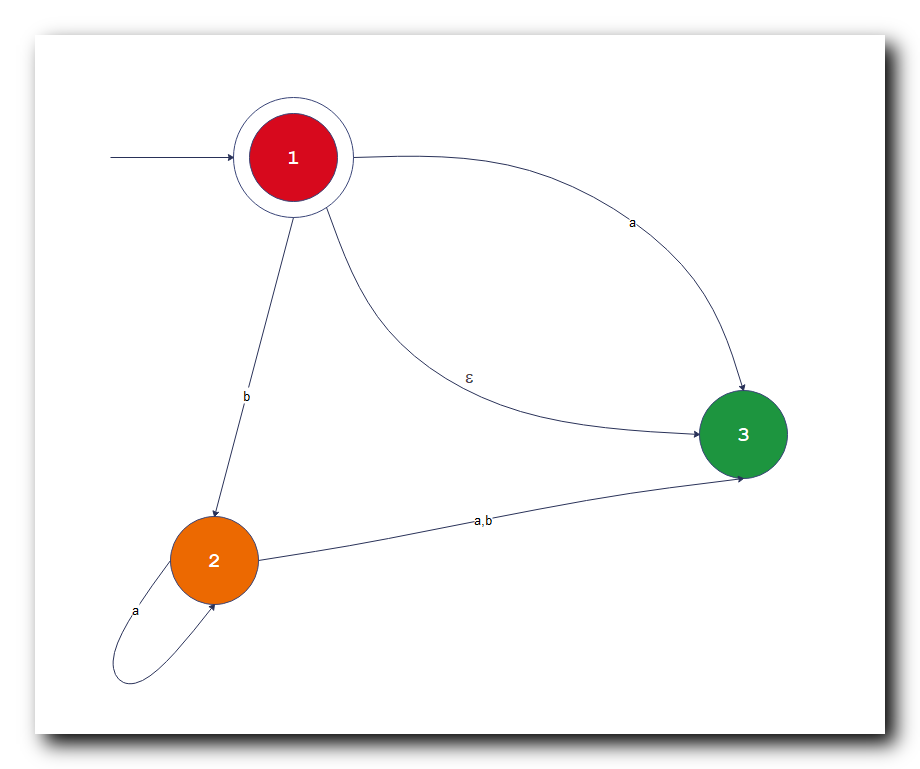上图绘制错误 , 暂时作废 , 看第一张图片 ;
字符集 :

Σ

=

{

a

,

b

}

\Sigma = \{a, b\}

将上述 非确定性有限自动机 ( NFA ) 转换成 确定性有限自动机 ( DFA ) , 需要将非确定性消除 , 只剩下确定性因素 ;

转换过程 使用特定算法实现 , 下面会详细描述该算法 ;

表格 : 绘制一个表格 , 表格列分别是

a

,

b

a, b

, 确定性有限自动机可以使用表格来表示 ;

开始状态分析 : 上述非确定性有限自动机开始状态

1

1

, 但是有一个

ε

\varepsilon

空字符 , 指向

3

3

状态 , 读取到空字符

ε

\varepsilon

后会无条件跳转到 后继状态

3

3

, 因此 该自动机有

2

2

个开始状态 ;
开始状态确定 :

{

1

,

3

}

\{1 , 3\}

, 将开始状态看做一个集合 , 将这两个状态组成的集合看做一个新的状态 ,

a

a

b

b

{

1

,

3

}

\{1, 3 \}

四、NFA 转 DFA ( 1 ) 开始状态 读取

a

a

字符 后继状态分析

a

a

b

b

{

1

,

3

}

\{1, 3 \}

表格 第

2

2

行 第

2

2

列 :

1 . 数值含义 : 该位置的含义是

{

1

,

3

}

\{1 , 3\}

开始状态下 , 读取 字符

a

a

之后的后续状态集 ;

2 . 后继状态分析 : 分别考虑

1

1

状态下读取

a

a

字符后的后继状态 ,

3

3

状态下读取

a

a

字符后的后继状态 ;

①

1

1

状态下读取

a

a

字符是空集

{

∅

}

\{ \varnothing \}

;
②

3

3

状态下读取

a

a

字符后继状态是

1

1

, 此时

1

1

状态又可以无条件跳转到

3

3

状态 , 可以与

1

1

状态并列 , 此时

3

3

状态 读取

a

a

字符的后继状态是

{

1

,

3

}

\{1, 3\}

;

3 . 后继状态取并集 : 将上述的 两个 后继状态

{

∅

}

\{ \varnothing \}

和

{

1

,

3

}

\{1, 3\}

, 取并集 , 就是

{

1

,

3

}

\{1 , 3\}

状态下读取

a

a

字符的后继状态 , 取并集的结果是

{

1

,

3

}

\{1, 3\}

;

第

2

2

行 第

2

2

列 的 值是

{

1

,

3

}

\{1, 3\}

, 代表

{

1

,

3

}

\{1 , 3\}

状态下读取 字符

a

a

, 后继状态是

{

1

,

3

}

\{1 , 3\}

状态 ;

a

a

b

b

{

1

,

3

}

\{1, 3 \}

{

1

,

3

}

\{1 , 3\}

五、NFA 转 DFA ( 2 ) 开始状态 读取

b

b

字符 后继状态分析

a

a

b

b

{

1

,

3

}

\{1, 3 \}

{

1

,

3

}

\{1 , 3\}

表格 第

2

2

行 第

3

3

列 :

1 . 数值含义 : 该位置的含义是

{

1

,

3

}

\{1 , 3\}

开始状态下 , 读取 字符

b

b

之后的后续状态集 ;

2 . 后继状态分析 : 分别考虑

1

1

状态下读取

b

b

字符后的后继状态 ,

3

3

状态下读取

b

b

字符后的后继状态 ;

①

1

1

状态下读取

b

b

字符 后继状态是

{

2

}

\{ 2 \}

;
②

3

3

状态下读取

b

b

字符后继状态是

{

∅

}

\{ \varnothing \}

空集 ;

3 . 后继状态取并集 : 将上述的 两个 后继状态

{

2

}

\{2\}

和

{

∅

}

\{ \varnothing \}

, 取并集 , 就是

{

1

,

3

}

\{1 , 3\}

状态下读取

b

b

字符的后继状态 , 取并集的结果是

{

2

}

\{2\}

;

第

2

2

行 第

3

3

列 的 值是

{

2

}

\{2\}

, 代表

{

1

,

3

}

\{1 , 3\}

状态下读取 字符

b

b

, 后继状态是

{

2

}

\{2\}

状态 ;

开始集合

{

1

,

3

}

\{1, 3 \}

, 读取

a

a

字符 , 读取

b

b

字符的后继状态 , 分析完毕 ;

a

a

b

b

{

1

,

3

}

\{1, 3 \}

{

1

,

3

}

\{1 , 3\}

{

2

}

\{2\}

六、NFA 转 DFA ( 3 ) 选取第二个状态进行分析

选取原则 : 没有出现过的状态 , 都要进行分析 ;
开始分析后续状态 , 第一个后继状态是

{

1

,

3

}

\{1,3\}

就是开始状态 , 已经分析过了 , 不再考虑 , 开始分析

{

2

}

\{2\}

状态 ;

a

a

b

b

{

1

,

3

}

\{1, 3 \}

{

1

,

3

}

\{1 , 3\}

{

2

}

\{2\}

{

2

}

\{2\}

七、NFA 转 DFA ( 4 )

2

2

状态读取

a

a

字符后续状态分析

a

a

b

b

{

1

,

3

}

\{1, 3 \}

{

1

,

3

}

\{1 , 3\}

{

2

}

\{2\}

{

2

}

\{2\}

表格 第

3

3

行 第

2

2

列 :

1 . 数值含义 : 该位置的含义是

{

2

}

\{2\}

开始状态下 , 读取 字符

a

a

之后的后续状态集 ;

2 . 后继状态分析 : 分别考虑

{

2

}

\{2\}

状态下读取

a

a

字符后的后继状态 是

{

2

,

3

}

\{2 , 3\}

;

3 . 后继状态 : 第

3

3

行 第

2

2

列 的 值是

{

2

,

3

}

\{2,3\}

, 代表

{

2

}

\{2\}

状态下读取 字符

a

a

, 后继状态是

{

2

,

3

}

\{2,3\}

状态 ;

a

a

b

b

{

1

,

3

}

\{1, 3 \}

{

1

,

3

}

\{1 , 3\}

{

2

}

\{2\}

{

2

}

\{2\}

{

2

,

3

}

\{2,3\}

八、NFA 转 DFA ( 5 )

2

2

状态读取

b

b

字符后续状态分析

a

a

b

b

{

1

,

3

}

\{1, 3 \}

{

1

,

3

}

\{1 , 3\}

{

2

}

\{2\}

{

2

}

\{2\}

{

2

,

3

}

\{2,3\}

表格 第

3

3

行 第

3

3

列 :

1 . 数值含义 : 该位置的含义是

{

2

}

\{2\}

开始状态下 , 读取 字符

b

b

之后的后续状态集 ;

2 . 后继状态分析 : 分别考虑

{

2

}

\{2\}

状态下读取

b

b

字符后的后继状态 是

{

3

}

\{ 3\}

;

3 . 后继状态 : 第

3

3

行 第

3

3

列 的 值是

{

3

}

\{3\}

, 代表

{

2

}

\{2\}

状态下读取 字符

b

b

, 后继状态是

{

3

}

\{3\}

状态 ;

a

a

b

b

{

1

,

3

}

\{1, 3 \}

{

1

,

3

}

\{1 , 3\}

{

2

}

\{2\}

{

2

}

\{2\}

{

2

,

3

}

\{2,3\}

{

3

}

\{3\}

九、NFA 转 DFA ( 6 ) 选取后续需要分析的状态

选取原则 : 没有出现过的状态 , 都要进行分析 ;

{

2

,

3

}

\{2,3\}

和

{

3

}

\{3\}

状态都是分析

{

2

}

\{2\}

状态的后继状态时新出现的状态 , 因此将这两个状态加入表格中 , 进行分析 ;

加入两个状态后 , 最新表格如下 :

a

a

b

b

{

1

,

3

}

\{1, 3 \}

{

1

,

3

}

\{1 , 3\}

{

2

}

\{2\}

{

2

}

\{2\}

{

2

,

3

}

\{2,3\}

{

3

}

\{3\}

{

2

,

3

}

\{2,3\}

{

3

}

\{3\}

下面开始分析后续内容 ;

十、NFA 转 DFA ( 7 )

{

2

,

3

}

\{2,3\}

状态 读取

a

a

字符 后继状态分析

a

a

b

b

{

1

,

3

}

\{1, 3 \}

{

1

,

3

}

\{1 , 3\}

{

2

}

\{2\}

{

2

}

\{2\}

{

2

,

3

}

\{2,3\}

{

3

}

\{3\}

{

2

,

3

}

\{2,3\}

{

3

}

\{3\}

表格 第

4

4

行 第

2

2

列 :

1 . 数值含义 : 该位置的含义是

{

2

,

3

}

\{2 , 3\}

开始状态下 , 读取 字符

a

a

之后的后续状态集 ;

2 . 后继状态分析 : 分别考虑

2

2

状态下读取

a

a

字符后的后继状态 ,

3

3

状态下读取

a

a

字符后的后继状态 ;

①

2

2

状态下读取

a

a

字符 后继状态是

{

2

,

3

}

\{ 2 , 3 \}

;
②

3

3

状态下读取

a

a

字符后继状态是

{

1

,

3

}

\{1 , 3\}

, 这里只要能到达状态

1

1

, 便需要无条件跳转到状态

3

3

, 因为

1

1

状态下读取空字符

ε

\varepsilon

即可转为

3

3

状态 , 因此

1

,

3

1,3

状态并列 ;

3 . 后继状态取并集 : 将上述的 两个 后继状态

{

2

,

3

}

\{2, 3\}

和

{

1

,

3

}

\{ 1,3 \}

, 取并集 , 就是

{

2

,

3

}

\{2 , 3\}

状态下读取

a

a

字符的后继状态 , 取并集的结果是

{

1

,

2

,

3

}

\{1,2,3\}

;

第

4

4

行 第

2

2

列 的 值是

{

1

,

2

,

3

}

\{1,2,3\}

, 代表

{

2

,

3

}

\{2 , 3\}

状态下读取 字符

a

a

, 后继状态是

{

1

,

2

,

3

}

\{1,2,3\}

状态 ;

a

a

b

b

{

1

,

3

}

\{1, 3 \}

{

1

,

3

}

\{1 , 3\}

{

2

}

\{2\}

{

2

}

\{2\}

{

2

,

3

}

\{2,3\}

{

3

}

\{3\}

{

2

,

3

}

\{2,3\}

{

1

,

2

,

3

}

\{1,2,3\}

{

3

}

\{3\}

十一、NFA 转 DFA ( 8 )

{

2

,

3

}

\{2,3\}

状态 读取

b

b

字符 后继状态分析

a

a

b

b

{

1

,

3

}

\{1, 3 \}

{

1

,

3

}

\{1 , 3\}

{

2

}

\{2\}

{

2

}

\{2\}

{

2

,

3

}

\{2,3\}

{

3

}

\{3\}

{

2

,

3

}

\{2,3\}

{

1

,

2

,

3

}

\{1,2,3\}

{

3

}

\{3\}

表格 第

4

4

行 第

3

3

列 :

1 . 数值含义 : 该位置的含义是

{

2

,

3

}

\{2 , 3\}

开始状态下 , 读取 字符

b

b

之后的后续状态集 ;

2 . 后继状态分析 : 分别考虑

2

2

状态下读取

b

b

字符后的后继状态 ,

3

3

状态下读取

b

b

字符后的后继状态 ;

①

2

2

状态下读取

b

b

字符 后继状态是

{

3

}

\{ 3 \}

;
②

3

3

状态下读取

b

b

字符后继状态是

{

∅

}

\{\varnothing\}

;

3 . 后继状态取并集 : 将上述的 两个 后继状态

{

3

}

\{3\}

和

{

∅

}

\{ \varnothing \}

, 取并集 , 就是

{

2

,

3

}

\{2 , 3\}

状态下读取

b

b

字符的后继状态 , 取并集的结果是

{

3

}

\{3\}

;

第

4

4

行 第

3

3

列 的 值是

{

3

}

\{3\}

, 代表

{

2

,

3

}

\{2 , 3\}

状态下读取 字符

b

b

, 后继状态是

{

3

}

\{3\}

状态 ;

a

a

b

b

{

1

,

3

}

\{1, 3 \}

{

1

,

3

}

\{1 , 3\}

{

2

}

\{2\}

{

2

}

\{2\}

{

2

,

3

}

\{2,3\}

{

3

}

\{3\}

{

2

,

3

}

\{2,3\}

{

1

,

2

,

3

}

\{1,2,3\}

{

3

}

\{3\}

{

3

}

\{3\}

十二、NFA 转 DFA ( 9 ) 选取后续需要分析的状态

选取原则 : 没有出现过的状态 , 都要进行分析 ;

{

1

,

2

,

3

}

\{1 ,2,3\}

是 新出现的状态 , 因此将这两个状态加入表格中 , 进行分析 ;
加入该状态后 , 最新表格如下 :

a

a

b

b

{

1

,

3

}

\{1, 3 \}

{

1

,

3

}

\{1 , 3\}

{

2

}

\{2\}

{

2

}

\{2\}

{

2

,

3

}

\{2,3\}

{

3

}

\{3\}

{

2

,

3

}

\{2,3\}

{

1

,

2

,

3

}

\{1,2,3\}

{

3

}

\{3\}

{

3

}

\{3\}

{

1

,

2

,

3

}

\{1,2,3\}

下面开始分析后续内容 ;

十三、NFA 转 DFA ( 10 )

{

3

}

\{3\}

状态 读取

a

a

字符 后继状态分析

a

a

b

b

{

1

,

3

}

\{1, 3 \}

{

1

,

3

}

\{1 , 3\}

{

2

}

\{2\}

{

2

}

\{2\}

{

2

,

3

}

\{2,3\}

{

3

}

\{3\}

{

2

,

3

}

\{2,3\}

{

1

,

2

,

3

}

\{1,2,3\}

{

3

}

\{3\}

{

3

}

\{3\}

{

1

,

2

,

3

}

\{1,2,3\}

表格 第

5

5

行 第

2

2

列 :

1 . 数值含义 : 该位置的含义是

{

3

}

\{ 3\}

开始状态下 , 读取 字符

a

a

之后的后续状态集 ;

2 . 后继状态分析 : 分别考虑

3

3

状态下读取

a

a

字符后的后继状态 是

{

1

,

3

}

\{1,3\}

3 . 后继状态 : 第

5

5

行 第

2

2

列 的 值是

{

1

,

3

}

\{1,3\}

, 代表

{

3

}

\{3\}

状态下读取 字符

a

a

, 后继状态是

{

1

,

3

}

\{1,3\}

状态 ;

a

a

b

b

{

1

,

3

}

\{1, 3 \}

{

1

,

3

}

\{1 , 3\}

{

2

}

\{2\}

{

2

}

\{2\}

{

2

,

3

}

\{2,3\}

{

3

}

\{3\}

{

2

,

3

}

\{2,3\}

{

1

,

2

,

3

}

\{1,2,3\}

{

3

}

\{3\}

{

3

}

\{3\}

{

1

,

3

}

\{1,3\}

{

1

,

2

,

3

}

\{1,2,3\}

十四、NFA 转 DFA ( 11 )

{

3

}

\{3\}

状态 读取

b

b

字符 后继状态分析

a

a

b

b

{

1

,

3

}

\{1, 3 \}

{

1

,

3

}

\{1 , 3\}

{

2

}

\{2\}

{

2

}

\{2\}

{

2

,

3

}

\{2,3\}

{

3

}

\{3\}

{

2

,

3

}

\{2,3\}

{

1

,

2

,

3

}

\{1,2,3\}

{

3

}

\{3\}

{

3

}

\{3\}

{

1

,

3

}

\{1,3\}

{

1

,

2

,

3

}

\{1,2,3\}

表格 第

5

5

行 第

3

3

列 :

1 . 数值含义 : 该位置的含义是

{

3

}

\{ 3\}

开始状态下 , 读取 字符

b

b

之后的后续状态集 ;

2 . 后继状态分析 : 分别考虑

3

3

状态下读取

b

b

字符后的后继状态 是

{

∅

}

\{\varnothing \}

3 . 后继状态 : 第

5

5

行 第

3

3

列 的 值是

{

∅

}

\{\varnothing \}

, 代表

{

3

}

\{3\}

状态下读取 字符

b

b

, 后继状态是

{

∅

}

\{\varnothing \}

状态 ;

a

a

b

b

{

1

,

3

}

\{1, 3 \}

{

1

,

3

}

\{1 , 3\}

{

2

}

\{2\}

{

2

}

\{2\}

{

2

,

3

}

\{2,3\}

{

3

}

\{3\}

{

2

,

3

}

\{2,3\}

{

1

,

2

,

3

}

\{1,2,3\}

{

3

}

\{3\}

{

3

}

\{3\}

{

1

,

3

}

\{1,3\}

{

∅

}

\{\varnothing \}

{

1

,

2

,

3

}

\{1,2,3\}

十五、NFA 转 DFA ( 12 )

{

1

,

2

,

3

}

\{1,2,3\}

状态 读取

a

a

字符 后继状态分析

a

a

b

b

{

1

,

3

}

\{1, 3 \}

{

1

,

3

}

\{1 , 3\}

{

2

}

\{2\}

{

2

}

\{2\}

{

2

,

3

}

\{2,3\}

{

3

}

\{3\}

{

2

,

3

}

\{2,3\}

{

1

,

2

,

3

}

\{1,2,3\}

{

3

}

\{3\}

{

3

}

\{3\}

{

1

,

3

}

\{1,3\}

{

∅

}

\{\varnothing \}

{

1

,

2

,

3

}

\{1,2,3\}

表格 第

6

6

行 第

2

2

列 :

1 . 数值含义 : 该位置的含义是

{

1

,

2

,

3

}

\{1,2 , 3\}

开始状态下 , 读取 字符

a

a

之后的后续状态集 ;

2 . 后继状态分析 : 分别考虑

1

1

状态下读取

a

a

字符后的后继状态 ,

2

2

状态下读取

a

a

字符后的后继状态 ,

3

3

状态下读取

a

a

字符后的后继状态 ;

①

1

1

状态下读取

a

a

字符 后继状态是

{

∅

}

\{ \varnothing \}

;
②

2

2

状态下读取

a

a

字符 后继状态是

{

2

,

3

}

\{ 2 , 3 \}

;
③

3

3

状态下读取

a

a

字符后继状态是

{

1

,

3

}

\{1 , 3\}

, 这里只要能到达状态

1

1

, 便需要无条件跳转到状态

3

3

, 因为

1

1

状态下读取空字符

ε

\varepsilon

即可转为

3

3

状态 , 因此

1

,

3

1,3

状态并列 ;

3 . 后继状态取并集 : 将上述的

3

3

个 后继状态

{

∅

}

\{ \varnothing \}

,

{

2

,

3

}

\{2, 3\}

和

{

1

,

3

}

\{ 1,3 \}

, 取并集 , 就是

{

1

,

2

,

3

}

\{1 , 2 , 3\}

状态下读取

a

a

字符的后继状态 , 取并集的结果是

{

1

,

2

,

3

}

\{1,2,3\}

;

第

6

6

行 第

2

2

列 的 值是

{

1

,

2

,

3

}

\{1,2,3\}

, 代表

{

1

,

2

,

3

}

\{1 , 2 , 3\}

状态下读取 字符

a

a

, 后继状态是

{

1

,

2

,

3

}

\{1,2,3\}

状态 ;

a

a

b

b

{

1

,

3

}

\{1, 3 \}

{

1

,

3

}

\{1 , 3\}

{

2

}

\{2\}

{

2

}

\{2\}

{

2

,

3

}

\{2,3\}

{

3

}

\{3\}

{

2

,

3

}

\{2,3\}

{

1

,

2

,

3

}

\{1,2,3\}

{

3

}

\{3\}

{

3

}

\{3\}

{

1

,

3

}

\{1,3\}

{

∅

}

\{\varnothing \}

{

1

,

2

,

3

}

\{1,2,3\}

{

1

,

2

,

3

}

\{1,2,3\}

十六、NFA 转 DFA ( 13 )

{

1

,

2

,

3

}

\{1,2,3\}

状态 读取

b

b

字符 后继状态分析

a

a

b

b

{

1

,

3

}

\{1, 3 \}

{

1

,

3

}

\{1 , 3\}

{

2

}

\{2\}

{

2

}

\{2\}

{

2

,

3

}

\{2,3\}

{

3

}

\{3\}

{

2

,

3

}

\{2,3\}

{

1

,

2

,

3

}

\{1,2,3\}

{

3

}

\{3\}

{

3

}

\{3\}

{

1

,

3

}

\{1,3\}

{

∅

}

\{\varnothing \}

{

1

,

2

,

3

}

\{1,2,3\}

{

1

,

2

,

3

}

\{1,2,3\}

表格 第

6

6

行 第

3

3

列 :

1 . 数值含义 : 该位置的含义是

{

1

,

2

,

3

}

\{1,2 , 3\}

开始状态下 , 读取 字符

b

b

之后的后续状态集 ;

2 . 后继状态分析 : 分别考虑

1

1

状态下读取

b

b

字符后的后继状态 ,

2

2

状态下读取

b

b

字符后的后继状态 ,

3

3

状态下读取

b

b

字符后的后继状态 ;

①

1

1

状态下读取

b

b

字符 后继状态是

{

2

}

\{ 2\}

;
②

2

2

状态下读取

a

a

字符 后继状态是

{

3

}

\{ 3 \}

;
③

3

3

状态下读取

a

a

字符后继状态是

{

∅

}

\{\varnothing\}

;

3 . 后继状态取并集 : 将上述的

3

3

个 后继状态

{

2

}

\{2\}

,

{

3

}

\{ 3 \}

和

{

∅

}

\{ \varnothing \}

, 取并集 , 就是

{

1

,

2

,

3

}

\{1 , 2 , 3\}

状态下读取

b

b

字符的后继状态 , 取并集的结果是

{

2

,

3

}

\{2,3\}

;

a

a

b

b

{

1

,

3

}

\{1, 3 \}

{

1

,

3

}

\{1 , 3\}

{

2

}

\{2\}

{

2

}

\{2\}

{

2

,

3

}

\{2,3\}

{

3

}

\{3\}

{

2

,

3

}

\{2,3\}

{

1

,

2

,

3

}

\{1,2,3\}

{

3

}

\{3\}

{

3

}

\{3\}

{

1

,

3

}

\{1,3\}

{

∅

}

\{\varnothing \}

{

1

,

2

,

3

}

\{1,2,3\}

{

1

,

2

,

3

}

\{1,2,3\}

{

2

,

3

}

\{2,3\}

十七、NFA 转 DFA ( 14 ) 结果分析

1 . 消除不确定性 : 下面的表格就是将 非确定性有限自动机 ( NFA ) 转为 确定性有限自动机 ( DFA ) 的结果 , 将状态集合当做一个新的状态 , 新状态由之前的 NFA 中的不同状态组合而来 , 由此消除不确定性 ;

a

a

b

b

{

1

,

3

}

\{1, 3 \}

{

1

,

3

}

\{1 , 3\}

{

2

}

\{2\}

{

2

}

\{2\}

{

2

,

3

}

\{2,3\}

{

3

}

\{3\}

{

2

,

3

}

\{2,3\}

{

1

,

2

,

3

}

\{1,2,3\}

{

3

}

\{3\}

{

3

}

\{3\}

{

1

,

3

}

\{1,3\}

{

∅

}

\{\varnothing \}

{

1

,

2

,

3

}

\{1,2,3\}

{

1

,

2

,

3

}

\{1,2,3\}

{

2

,

3

}

\{2,3\}

2 . 定义开始状态 : 将

{

1

,

3

}

\{1, 3 \}

定义成开始状态 ;

3 . 定义接收状态 : 原来的 非确定性有限自动机 ( NFA ) 中

1

1

是接受状态 , 在新的 确定性有限自动机 ( DFA ) 中 , 只要状态集合中包含

1

1

, 那么该状态集合就是 接受状态 , 因此这里

{

1

,

3

}

\{1, 3 \}

和

{

1

,

2

,

3

}

\{1,2,3\}

两个状态集 代表的 新状态 是接受状态 ;
展开全文计算理论
• 一、正则表达式转为非确定性有限自动机 NFA 要点、 二、正则表达式转为非确定性有限自动机 NFA 示例 1、 三、正则表达式转为非确定性有限自动机 NFA 示例 2、 四、正则表达式转为非确定性有限自动机 NFA 示例 3


文章目录
一、正则表达式转为非确定性有限自动机 NFA 要点二、正则表达式转为非确定性有限自动机 NFA 示例 1三、正则表达式转为非确定性有限自动机 NFA 示例 2四、正则表达式转为非确定性有限自动机 NFA 示例 3

一、正则表达式转为非确定性有限自动机 NFA 要点

正则表达式转为非确定性有限自动机 NFA 流程 :
① 原子自动机 : 首先要构造 原子自动机 , 从 非接受状态 指向 接受状态 ;
② 基本拼装 : 将原子自动机进行 基本的拼装 , 并运算 , 交运算 ;
③ 复杂拼装 : 将 基本拼装的自动机 进行 进一步拼装 ;

拼装原则 : 使用

ε

\varepsilon

箭头 进行拼装 ;
① 串联 : 前者的接受状态 使用

ε

\varepsilon

箭头 指向 后者的开始状态 , 前者接受状态取消 ; 如果有两个接受状态 , 那么就需要引出两个箭头
② 并联 : 在二者之前 , 重新添加一个非接受状态的开始状态 , 使用两个

ε

\varepsilon

箭头 分别指向二者的开始状态 ;
③ 星运算 : 重新添加一个接受状态的开始状态 , 使用

ε

\varepsilon

箭头从 接受状态 指向 开始状态 ; 注意所有的接受状态 , 都要使用

ε

\varepsilon

箭头指向开始状态 ;

二、正则表达式转为非确定性有限自动机 NFA 示例 1

将正则表达式

(

0

∪

1

)

∗

000

(

0

∪

1

)

∗

\rm (0 \cup 1)^*000 ( 0 \cup 1 )^*

转为 NFA ;

构造原子自动机 : 注意从 非接受状态

→

\to

接受状态 ;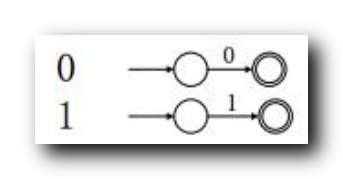(

0

∪

1

)

\rm (0 \cup 1)

并联拼装 : 在二者前面添加 非接受状态 起始状态 ;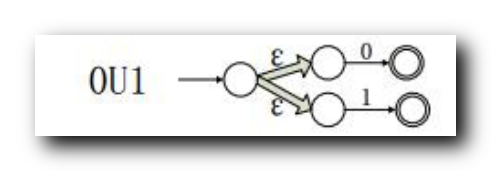(

0

∪

1

)

∗

\rm (0 \cup 1)^*

星运算 : 使 接受状态

→

\to

起始状态 , 并添加一个 接受状态 起始状态 , 指向原来的起始状态 ;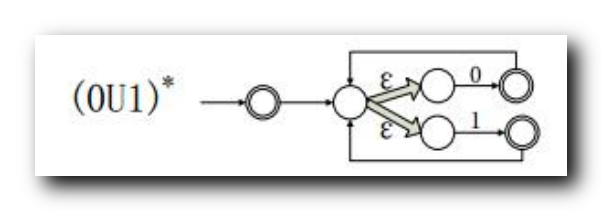000

000

串联 : 前者的接受状态 必须转为 非接受状态 , 前者的接受状态 必须转为 非接受状态 , 后者的状态是不变的 ;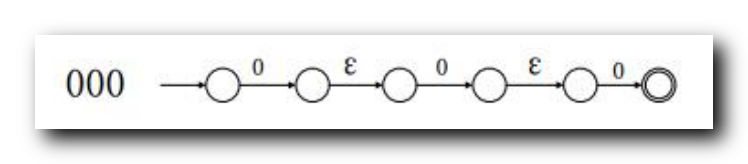总拼装 :
串联注意事项 :

(

0

∪

1

)

∗

\rm (0 \cup 1)^*

有

3

3

个接受状态 , 需要引出

3

3

个

ε

\varepsilon

箭头 指向

000

000

代表的自动机 的 开始状态 ;
串联时的状态改变 : 使用

ε

\varepsilon

箭头 连接 前者的接受状态

→

\to

后者的起始状态;
串联时 前者的接受状态 必须转为 非接受状态 ,
后者的状态是不变的 , 如果是接受状态 , 那么就保持接受状态不变 , 同理如果是非接受状态 , 那么保持非接受状态不变 ;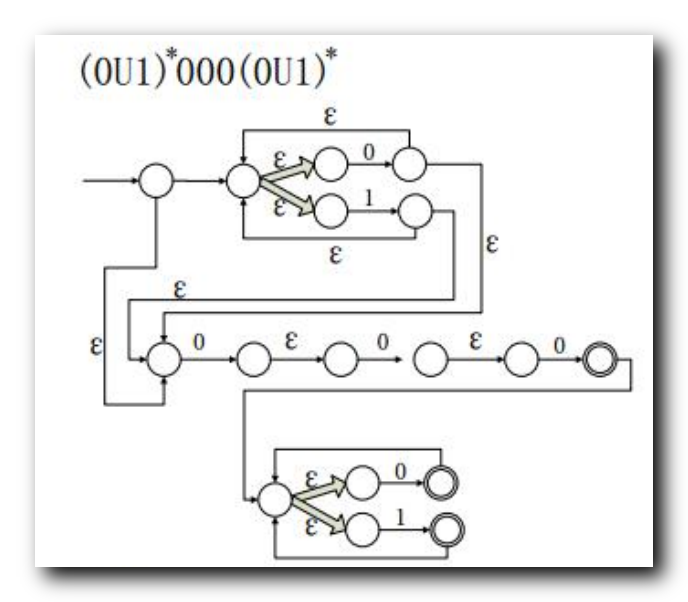化简后结果 :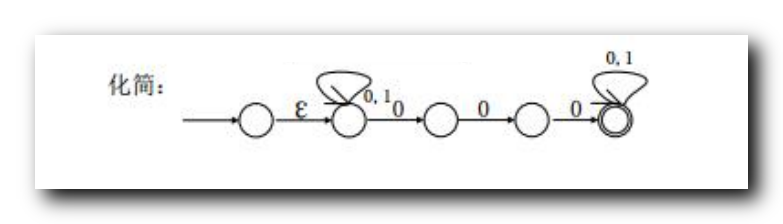三、正则表达式转为非确定性有限自动机 NFA 示例 2

将正则表达式

(

(

(

00

)

∗

(

11

)

)

∪

01

)

∗

\rm ( ( (00) ^* (11) ) \cup 01 )^*

转为 NFA ;

构造原子自动机 : 注意从 非接受状态

→

\to

接受状态 ;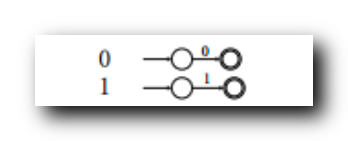00

00

串联 : 前者的接受状态 必须转为 非接受状态 , 前者的接受状态 必须转为 非接受状态 , 后者的状态是不变的 ;(

00

)

∗

(00)^*

星运算 : 使 接受状态

→

\to

起始状态 , 并添加一个 接受状态 起始状态 , 指向原来的起始状态 ;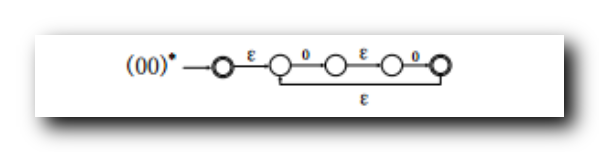11

11

串联 : 前者的接受状态 必须转为 非接受状态 , 前者的接受状态 必须转为 非接受状态 , 后者的状态是不变的 ;(

(

00

)

∗

(

11

)

)

((00)^* (11))

串联 : 前者的接受状态 必须转为 非接受状态 , 前者的接受状态 必须转为 非接受状态 , 后者的状态是不变的 ;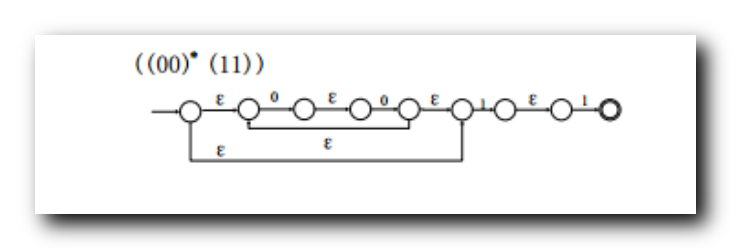(

(

00

)

∗

(

11

)

)

∪

01

((00)^* (11)) \cup 01

并联 : 在二者前面添加 非接受状态 起始状态 ;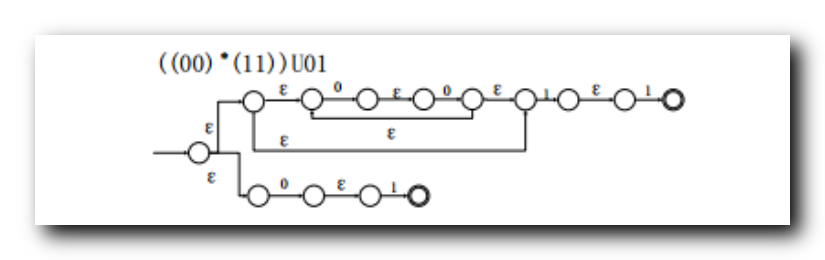(

(

(

00

)

∗

(

11

)

)

∪

01

)

∗

(((00)^* (11)) \cup 01)^*

星运算 : 使 接受状态

→

\to

起始状态 , 并添加一个 接受状态 起始状态 , 指向原来的起始状态 ;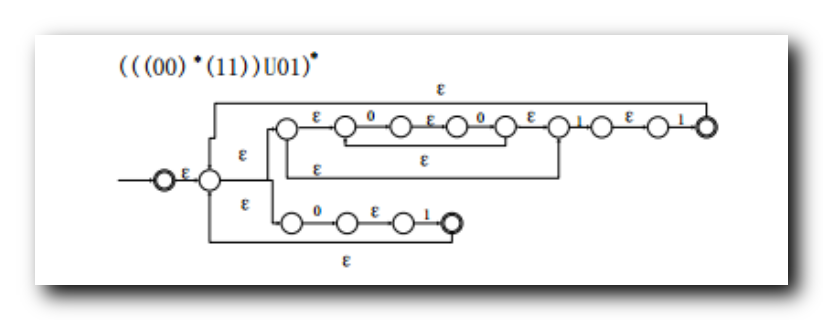化简后结果 :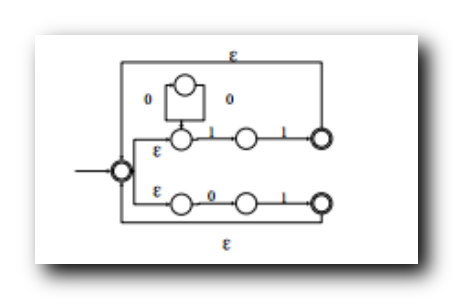四、正则表达式转为非确定性有限自动机 NFA 示例 3

将正则表达式

∅

∗

\varnothing ^*

转为 NFA ;

∅

∗

\varnothing ^*

=

{

ε

}

=\{ \varepsilon \}

构造原子自动机 : 注意从 非接受状态

→

\to

接受状态 ;展开全文正则表达式 自动机 计算理论
• 整理一下上学期写的代码，...NFA相关： 利用五元组，创建NFA模型 输入字符串，判定是否被该NFA模型接受 DFA相关： 利用五元组，创建DFA模型 输入字符串，判断是否被该DFA模型接受 NFA转DFA DFA最小化 RL与DFA转换算法 python 确定有穷自动机
• NFA到DFA转换（确定） DFA最小化 DFA解决方案计数 足协平等 FA超集/子集测试 FA适当的超集/子集测试 英足总有一个有效的解决方案 FA打印 FA导出为DOT FA字符串匹配 FA交叉路口 足总工会 FA否定 FA反向 FA到Regex的...PHP
• 　在编译系统中，词法分析阶段是整个编译系统的基础。对于单词的识别，有限自动机FA是一种十分有效的工具。有限自动机由其映射f是否为单值而分为确定的有限自动机DFA和确定的有限自动机NFA。...这种不确定性给...
• 一个使用Thompson构造将正则表达式转换为非确定性有限自动机（NFA）的c ++程序。 此外，它被简化为确定性有限自动机（DFA），并且有一个函数可用于检查属于给定正则表达式的各种字符串。 做得更好:) PS：-不久将...C++
• | 确定性有限自动机 转为 正则表达式 ) 三、根据正则表达式构造自动机 根据下面的 正则表达式 构造 非确定性有限自动机 ( NFA ) , 刚好能 识别上述正则表达式表示的语言 ; ( a b ∪ a ) ∗ ( ab \cup a )^* (ab∪a)...正则语言 正则表达式
• ## NFA的确定化

万次阅读 2017-12-01 09:44:45
实验一 NFA确定化 一、实验目的 1.通过本次实验，加深对正则表达式、NFA、DFA及其识别的语言的理解; 2.掌握从NFA到DFA的转换，以及用子集法把NFA转换成DFA理论，编程实现将NFA(不确定有穷自动机)转换为...编译原理
• 一个方便的工具，可以可视化将正则表达式（RE）转换为不确定性自动机（NFA），确定性自动机（DFA）和最小化确定性自动机（min-DFA）的过程。 要求 Graphviz Networkx matplotlib 目的 更深入地了解生成NFA ， DFA...Python
• 一、非确定性有限自动机的接受问题、 二、证明 "非确定性有限自动机的接受问题" 可判定性、图灵机
• NFA确定化，即将NFA转化为DFA。本文档拥有NFA TO DFA的详细过程，包括相关概念介绍，算法思想，实现代码，运行例证，运行截屏等。如果你也要写同题目的课程设计，你可以参考本文档。由于文档针对强，所以资源分...
• - NFA确定化，Github代码地址 - DFA的最小化，Github代码地址 上课第二周左右，谢红侠老师布置了第一次加平时分作业，期间积极并不高，虽然一直挂念着，最后还是拖到了9月底才完成。不过全部算法和内容皆是自己....c++ 编译原理 自动机
• NFA 确定化为 DFA 1实验目的 设计并实现将 NFA 确定化为 DFA 的子集构造算法 从而更好地理解有限自动机之间的 等价掌握词法分析器自动产生器的构造技术该算法也是构造 LR 分析器的基础 2实验要求 设计并实现计算...
• 一个非确定的有穷自动机(NFA)M是一个五元式： M=(S,∑,δ,S0,F) S是一个有限集，它额每个元素称为一个状态。∑是一个有穷字母表，它的每个元素称为一个输入字符δ是一个从S×∑∗至S子集额单值映射。即：δ:...
• 将上下文无关文法读入内存之后，可以将它转换成非确定有限状态自动机。当然，不是所有的上下文无关文法都能够转换成自动机的，前提条件是这个上下文无关文法能够与正则定义等价。因此，在进行转换之前，我们需要先...上下文无关文法 自动机
• 编译原理实验二 NFA确定化与DFA最小化一、实验目的二、实验任务三、实验内容1.NFA确定化2.DFA最小化四、实验准备1.NFA、DFA的存储格式2.测试样例的选择3.文件存储格式（以第三个样例为例）五、实验设计1.NFA确定化...
• 正规式-&gt;最小化DFA说明 整体的步骤是三步： 一，先把正规式转换为NFA（确定有穷自动机）, 二，在把NFA通过“子集构造法”转化为DFA， 三，在把DFA通过“分割法”... 同样的例题，把转换好的NFA确定化...
• 一个非确定的有穷自动机(NFA)M是一个五元式： M=(S,∑,δ,S0,F)  S是一个有限集，它额每个元素称为一个状态。∑是一个有穷字母表，它的每个元素称为一个输入字符δ是一个从S×∑∗至S子集额单值映射。即：δ:...编译原理
• 文章目录推广型的非确定性有限自动机 ( GNFA ) 引入推广型的非确定性有限自动机 ( GNFA ) 删除状态确定性有限自动机 ( DFA ) 转为 正则表达式确定性有限自动机 ( DFA ) 转为 正则表达式 ( 1 ) 添加开始状态 SSS 和...计算理论 正则语言 自动机 正则表达式
• 对于每个NFA M存在一个DFA M’，使得L(M)=L(M’)--------等价证明，NFA确定化 假定NFA M=<S, Σ, δ, S 0 , F>，我们对M的状态转换图进行以下改造： 解决初始状态唯一：引进新的初态结点X和终态结点Y，...
• 三、实验内容（1）确定NFA与DFA的存储格式。 要求为3个以上测试NFA准备好相应有限自动机的存储文件。（可利用实验一（二）的基础） （2）用C或JAVA语言编写将NFA转换成DFA的子集构造法的程序。 （3）测试验证程
• 非确定性有穷自动机nondeterministic finite automation。 ε-转换ε-transition 是无需考虑输入串（且无需消耗任何字符）就有可能发声的转换，它可看作是一个空串的“匹配”。 转换表transition table 是一个 T...
• 第一部分 NFA确定化 一、实验目的 学习和掌握将NFA转为DFA的子集构造法。 二、实验任务 （1）存储NFA与DFA； （2）编程实现子集构造法将NFA转换成DFA。 三、实验内容 （1）确定NFA与DFA的存储格式。 要求为3个...
• SwiftNFA 是一种在 Swift 中实现非确定性有限自动机的方法。 ###Usage SwiftNFA 有一个称为NFA的主要泛型类，它代表自动机。 您可以使用NFA(initialState: iState, acceptingStates: aStates)实例化自动机，其中...Swift
• 输入：非确定有穷状态自动机NFA 输出：确定化的有穷状态自动机DFA
• ：状态s和t必须同时为终态或终态  ·   蔓延条件 ：对于所有输入符号，状态s和状态t必须转化到等价的状态里    接下来是关于如何利用上面的两个条件来判断是否为等价状态，来一张复杂的状态转换图，是不是......